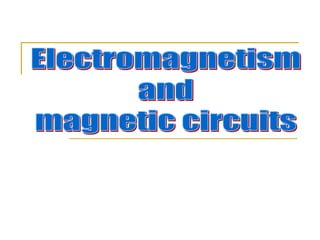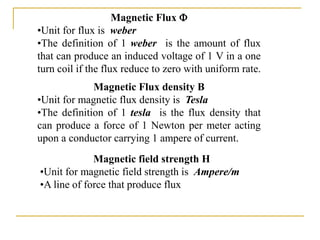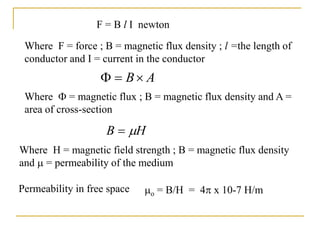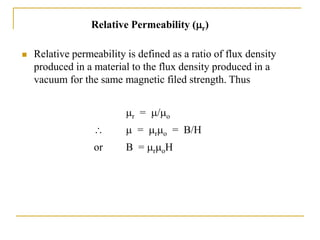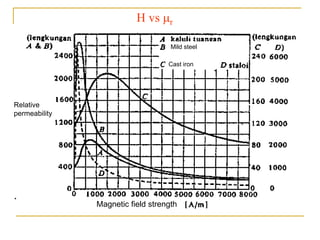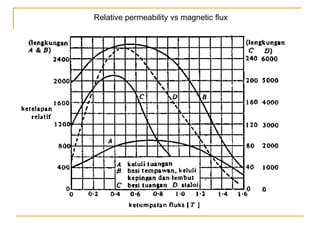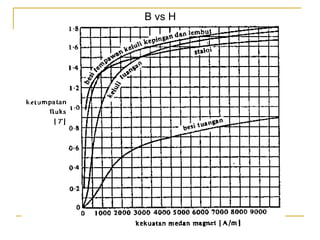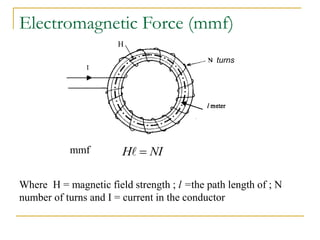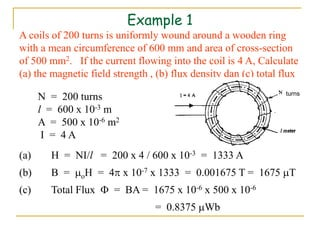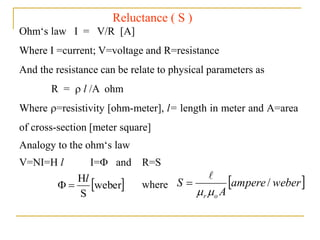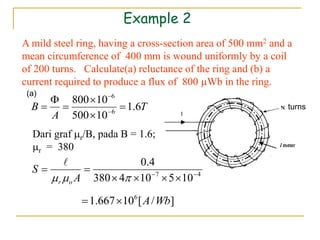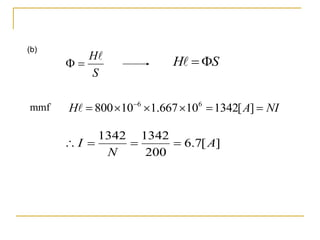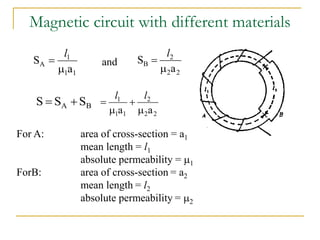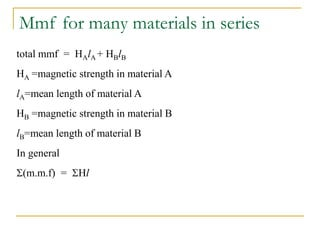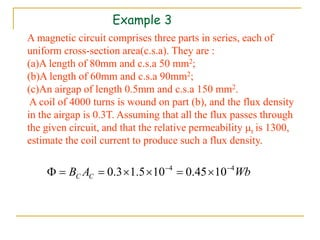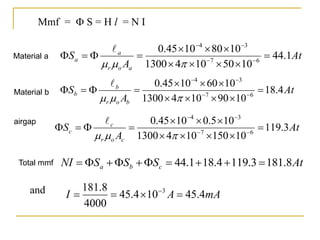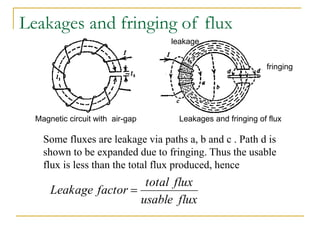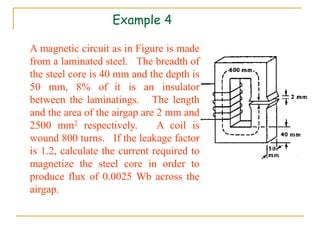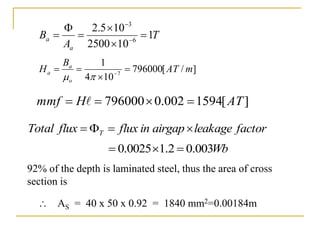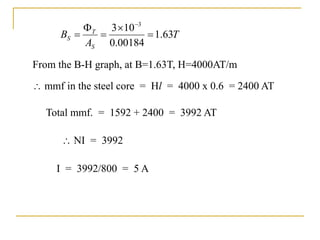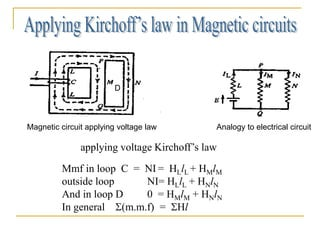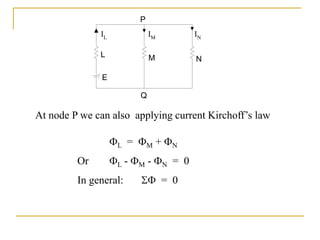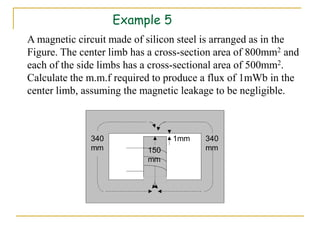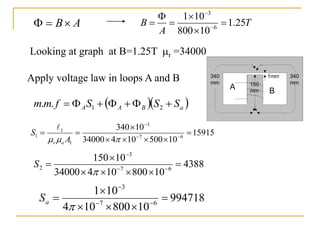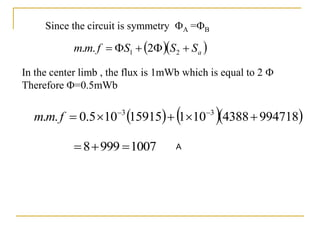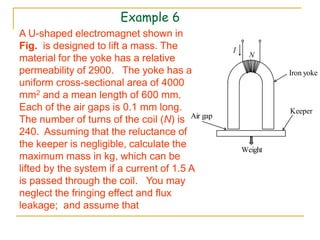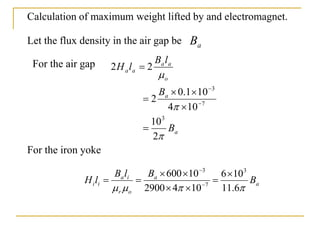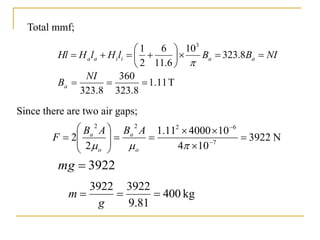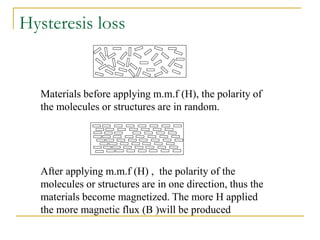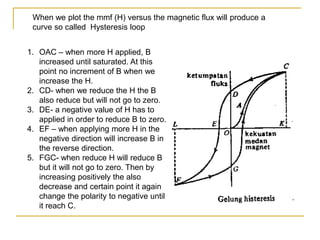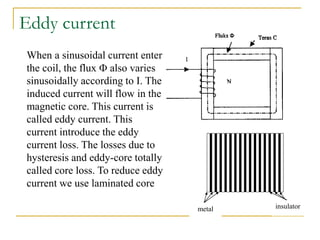1 of 31

### 11 magnetic circuit (1).ppt

1. Magnetic Flux density B •Unit for magnetic flux density is Tesla •The definition of 1 tesla is the flux density that can produce a force of 1 Newton per meter acting upon a conductor carrying 1 ampere of current. Magnetic Flux  •Unit for flux is weber •The definition of 1 weber is the amount of flux that can produce an induced voltage of 1 V in a one turn coil if the flux reduce to zero with uniform rate. Magnetic field strength H •Unit for magnetic field strength is Ampere/m •A line of force that produce flux
2. F = B l I newton Where F = force ; B = magnetic flux density ; l =the length of conductor and I = current in the conductor A B   Where  = magnetic flux ; B = magnetic flux density and A = area of cross-section H B   Where H = magnetic field strength ; B = magnetic flux density and  = permeability of the medium o = B/H = 4 x 10-7 H/m Permeability in free space
3.  Relative permeability is defined as a ratio of flux density produced in a material to the flux density produced in a vacuum for the same magnetic filed strength. Thus Relative Permeability (r) r = /o   = ro = B/H or B = roH
4. H vs r Relative permeability Magnetic field strength Cast iron Mild steel
5. Relative permeability vs magnetic flux
6. B vs H
7. Electromagnetic Force (mmf) turns H NI H   mmf Where H = magnetic field strength ; l =the path length of ; N number of turns and I = current in the conductor
8. Example 1 A coils of 200 turns is uniformly wound around a wooden ring with a mean circumference of 600 mm and area of cross-section of 500 mm2. If the current flowing into the coil is 4 A, Calculate (a) the magnetic field strength , (b) flux density dan (c) total flux N = 200 turns l = 600 x 10-3 m A = 500 x 10-6 m2 I = 4 A (a) H = NI/l = 200 x 4 / 600 x 10-3 = 1333 A (b) B = oH = 4 x 10-7 x 1333 = 0.001675 T = 1675 T (c) Total Flux  = BA = 1675 x 10-6 x 500 x 10-6 = 0.8375 Wb turns
9. Ohm‘s law I = V/R [A] Where I =current; V=voltage and R=resistance And the resistance can be relate to physical parameters as R =  l /A ohm Where =resistivity [ohm-meter], l= length in meter and A=area of cross-section [meter square] Analogy to the ohm‘s law V=NI=H l I= and R=S Reluctance ( S )   weber S Hl     weber ampere A S o r /     where
10. Example 2 A mild steel ring, having a cross-section area of 500 mm2 and a mean circumference of 400 mm is wound uniformly by a coil of 200 turns. Calculate(a) reluctance of the ring and (b) a current required to produce a flux of 800 Wb in the ring. T A B 6 . 1 10 500 10 800 6 6         Dari graf r/B, pada B = 1.6; r = 380 turns 4 7 10 5 10 4 380 4 . 0            A S o r  ] / [ 10 667 . 1 6 Wb A   (a)
11. (b) S H   S H    NI A H        ] [ 1342 10 667 . 1 10 800 6 6  mmf ] [ 7 . 6 200 1342 1342 A N I    
12. Magnetic circuit with different materials 1 1 1 A a S   l 2 2 2 B a S   l B A S S S   2 2 2 1 1 1 a a     l l and For A: area of cross-section = a1 mean length = l1 absolute permeability = 1 ForB: area of cross-section = a2 mean length = l2 absolute permeability = 2
13. Mmf for many materials in series total mmf = HAlA + HBlB HA =magnetic strength in material A lA=mean length of material A HB =magnetic strength in material B lB=mean length of material B In general (m.m.f) = Hl
14. Example 3 A magnetic circuit comprises three parts in series, each of uniform cross-section area(c.s.a). They are : (a)A length of 80mm and c.s.a 50 mm2; (b)A length of 60mm and c.s.a 90mm2; (c)An airgap of length 0.5mm and c.s.a 150 mm2. A coil of 4000 turns is wound on part (b), and the flux density in the airgap is 0.3T. Assuming that all the flux passes through the given circuit, and that the relative permeability r is 1300, estimate the coil current to produce such a flux density. Wb A B C C 4 4 10 45 . 0 10 5 . 1 3 . 0         
15. mA A I 4 . 45 10 4 . 45 4000 8 . 181 3      At A S a o r a a 1 . 44 10 50 10 4 1300 10 80 10 45 . 0 6 7 3 4                     At S S S NI c b a 8 . 181 3 . 119 4 . 18 1 . 44           and Mmf =  S = H l = N I At A S b o r b b 4 . 18 10 90 10 4 1300 10 60 10 45 . 0 6 7 3 4                     At A S c o r c c 3 . 119 10 150 10 4 1300 10 5 . 0 10 45 . 0 6 7 3 4                     Material a Material b airgap Total mmf
16. Leakages and fringing of flux Some fluxes are leakage via paths a, b and c . Path d is shown to be expanded due to fringing. Thus the usable flux is less than the total flux produced, hence Magnetic circuit with air-gap Leakages and fringing of flux flux usable flux total factor Leakage  fringing leakage
17. Example 4 A magnetic circuit as in Figure is made from a laminated steel. The breadth of the steel core is 40 mm and the depth is 50 mm, 8% of it is an insulator between the laminatings. The length and the area of the airgap are 2 mm and 2500 mm2 respectively. A coil is wound 800 turns. If the leakage factor is 1.2, calculate the current required to magnetize the steel core in order to produce flux of 0.0025 Wb across the airgap.
18. T A B a a 1 10 2500 10 5 . 2 6 3         ] / [ 796000 10 4 1 7 m AT B H o a a        factor leakage airgap in flux flux Total T     ] [ 1594 002 . 0 796000 AT H mmf      Wb 003 . 0 2 . 1 0025 . 0    92% of the depth is laminated steel, thus the area of cross section is  AS = 40 x 50 x 0.92 = 1840 mm2=0.00184m
19. T A B S T S 63 . 1 00184 . 0 10 3 3       From the B-H graph, at B=1.63T, H=4000AT/m  mmf in the steel core = Hl = 4000 x 0.6 = 2400 AT Total mmf. = 1592 + 2400 = 3992 AT I = 3992/800 = 5 A  NI = 3992
20. Mmf in loop C = NI = HLlL + HMlM outside loop NI= HLlL + HNlN And in loop D 0 = HMlM + HNlN In general (m.m.f) = Hl Magnetic circuit applying voltage law Analogy to electrical circuit D applying voltage Kirchoff’s law
21. L = M + N Or L - M - N = 0 In general:  = 0 At node P we can also applying current Kirchoff’s law P L M N E Q IL IN IM
22. Example 5 340 mm 340 mm 150 mm 1mm A magnetic circuit made of silicon steel is arranged as in the Figure. The center limb has a cross-section area of 800mm2 and each of the side limbs has a cross-sectional area of 500mm2. Calculate the m.m.f required to produce a flux of 1mWb in the center limb, assuming the magnetic leakage to be negligible.
23.    a B A A S S S f m m        2 1 . . A B   T A B 25 . 1 10 800 10 1 6 3         15915 10 500 10 4 34000 10 340 6 7 3 1 1 1               A S o r  Looking at graph at B=1.25T r =34000 Apply voltage law in loops A and B 340 mm 340 mm 150 mm 1mm A B 4388 10 800 10 4 34000 10 150 6 7 3 2            S 994718 10 800 10 4 10 1 6 7 3           a S
24. 1007 999 8         994718 4388 10 1 15915 10 5 . 0 . . 3 3        f m m Since the circuit is symmetry A =B In the center limb , the flux is 1mWb which is equal to 2  Therefore =0.5mWb    a S S S f m m      2 1 2 . . A
25. N I Weight Iron yoke Keeper Air gap Example 6 A U-shaped electromagnet shown in Fig. is designed to lift a mass. The material for the yoke has a relative permeability of 2900. The yoke has a uniform cross-sectional area of 4000 mm2 and a mean length of 600 mm. Each of the air gaps is 0.1 mm long. The number of turns of the coil (N) is 240. Assuming that the reluctance of the keeper is negligible, calculate the maximum mass in kg, which can be lifted by the system if a current of 1.5 A is passed through the coil. You may neglect the fringing effect and flux leakage; and assume that
26. a B a a o a a a a B B l B l H    2 10 10 4 10 1 . 0 2 2 2 3 7 3         a a o r i a i i B B l B l H     6 . 11 10 6 10 4 2900 10 600 3 7 3           Calculation of maximum weight lifted by and electromagnet. Let the flux density in the air gap be For the air gap For the iron yoke
27. NI B B l H l H Hl a a i i a a              8 . 323 10 6 . 11 6 2 1 3  T 11 . 1 8 . 323 360 8 . 323    NI Ba N 3922 10 4 10 4000 11 . 1 2 2 7 6 2 2 2                     o a o a A B A B F 3922  mg kg 400 81 . 9 3922 3922    g m Total mmf; Since there are two air gaps;
28. Hysteresis loss Materials before applying m.m.f (H), the polarity of the molecules or structures are in random. After applying m.m.f (H) , the polarity of the molecules or structures are in one direction, thus the materials become magnetized. The more H applied the more magnetic flux (B )will be produced
29. When we plot the mmf (H) versus the magnetic flux will produce a curve so called Hysteresis loop 1. OAC – when more H applied, B increased until saturated. At this point no increment of B when we increase the H. 2. CD- when we reduce the H the B also reduce but will not go to zero. 3. DE- a negative value of H has to applied in order to reduce B to zero. 4. EF – when applying more H in the negative direction will increase B in the reverse direction. 5. FGC- when reduce H will reduce B but it will not go to zero. Then by increasing positively the also decrease and certain point it again change the polarity to negative until it reach C.
30. Eddy current metal insulator When a sinusoidal current enter the coil, the flux  also varies sinusoidally according to I. The induced current will flow in the magnetic core. This current is called eddy current. This current introduce the eddy current loss. The losses due to hysteresis and eddy-core totally called core loss. To reduce eddy current we use laminated core